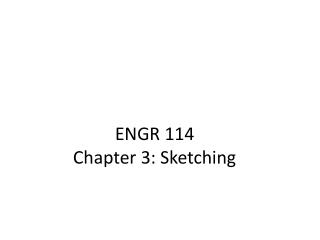# ENGR 114 Chapter 3: Sketching - PowerPoint PPT PresentationDownload PresentationENGR 114 Chapter 3: Sketching

ENGR 114 Chapter 3: Sketching
Download Presentation## ENGR 114 Chapter 3: Sketching

- - - - - - - - - - - - - - - - - - - - - - - - - - - E N D - - - - - - - - - - - - - - - - - - - - - - - - - - -
##### Presentation Transcript

1. ENGR 114 Chapter 3: Sketching

2. Sketching (Freehand) • Sketching is a method of visualizing and conceptualizing your ideas that will allow you to communicate your ideas to others. • Sketching is not intended to produced final engineering drawings, but it is an important step in the engineering design process.

3. A great idea comes to life!

4. Sketching Pencils • Pencils come with leads of varying degrees of hardness. • Hard grades H – 4H • Soft grades B – 4B • HB = #2 Pencil • The larger the number the harder of softer the lead.

5. Understanding Sketching Techniques • Analyzing complex objects • The ability to break down complex shapes into simpler geometric primitives is an essential skill for sketching and modeling objects • Basic curves and straight lines are the basis of many objects

6. Understanding Sketching Techniques • Maintaining Proportions – It is very important to keep the sketch in proportion • It is beneficial to block out general proportions using construction lines.

7. Understanding Sketching Techniques • Essential shapes can be blocked in using construction lines • Proportions are also maintained

8. Sketching Lines • Straight Lines • Angle Paper • Swing from elbow and not wrist • Draw in one fluid stroke • Experiment with direction

9. Sketching Circles

10. Sketching Arcs

11. Practice Sketch – Wood Plane

12. Sketching Objects (3D) • Viewpoint • You should always maintain the same viewpoint when sketching an object. • Isometric Sketching • When sketching 3D objects it is easiest to choose a viewpoint that will produce an isometric sketch.

13. Basic Structure of Objects • Cube or Rectangular Solid • Cylinder • Cone / Pyramid • Sphere • Combination of the above

14. Sketching Cubes or Rectangular Solids

15. Sketching Cylinders, Cones and Pyramids

16. Sketching Spheres

17. Combination of Shapes

18. Geometric Constructions • We are going to cover five basic geometric constructions that will be useful in drawing. • Bisect an angle • Bisecting a line using a compass. • Draw a perpendicular to a line at a point. • Finding the center of a circle • Dividing a line into n equal segments

19. Bisect an angle

20. Bisecting a line using a compass

21. Draw a perpendicular to a line at a point

22. Finding the center of a circle

23. Dividing a line into n equal segments